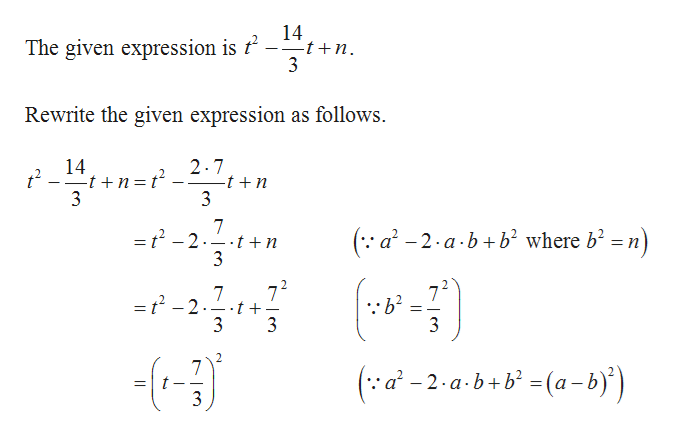# Find the value of n so that the expression is a perfect square trinomial and thenfactor the trinomial.t2 - 14/3 t + n

Question
6 views

Find the value of n so that the expression is a perfect square trinomial and thenfactor the trinomial.

t2 - 14/3 t + n

check_circle

Step 1help_outlineImage Transcriptionclose14 The given expression is t –÷t+n. 3 Rewrite the given expression as follows. 2.7 -t +n 3 14 t -t +n=t 3 (:: a' - 2-a-b+b² where b² = n) =t -2.- t +n 3 72 =t -2..t+. ::b? = 3 3 3 · a² – 2 · a ·b+ b² =(a - b)') 3 2. fullscreen

### Want to see the full answer?

See Solution

#### Want to see this answer and more?

Solutions are written by subject experts who are available 24/7. Questions are typically answered within 1 hour.*

See Solution
*Response times may vary by subject and question.
Tagged in

### Other